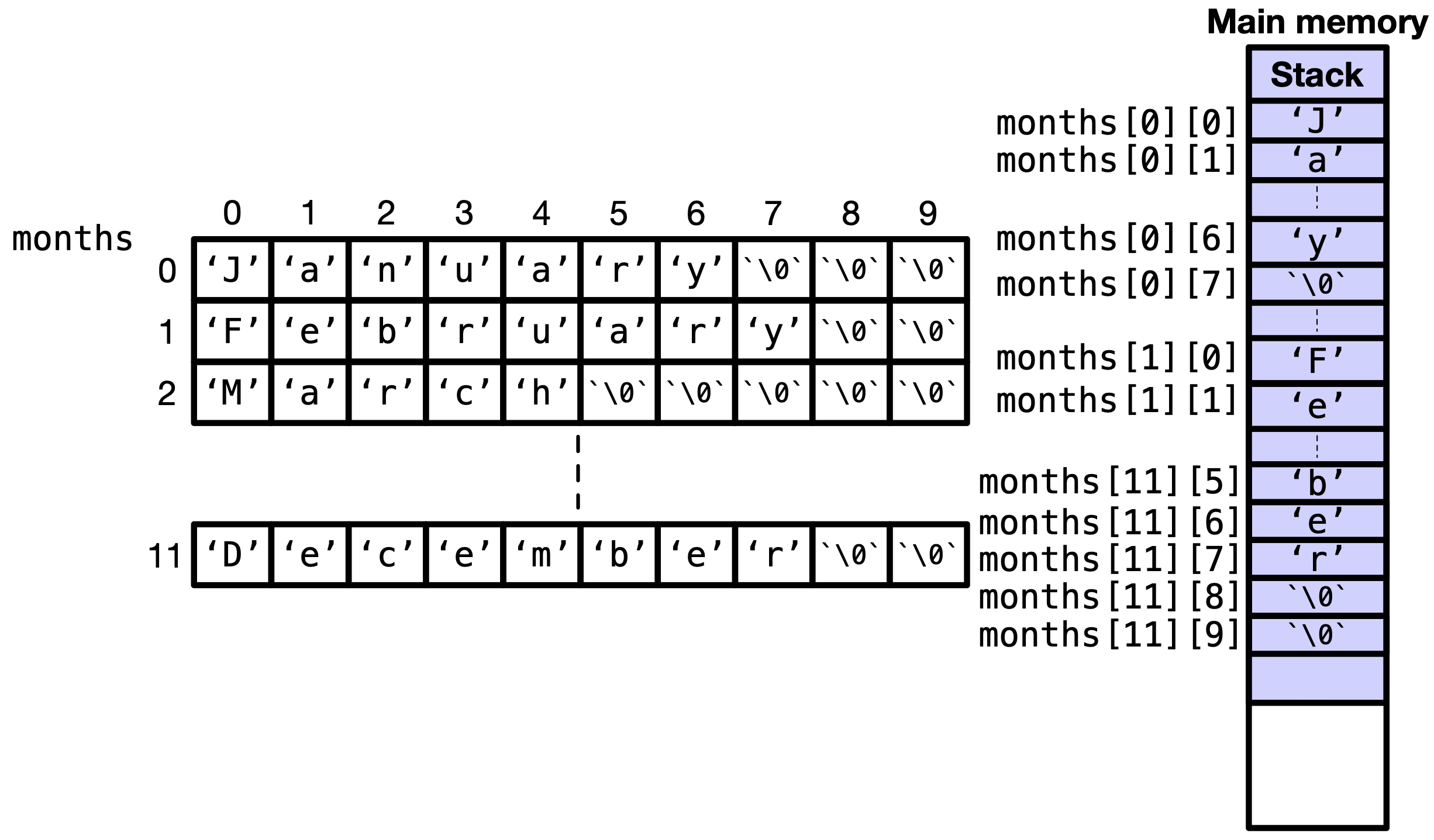# 10.4. Array of Strings#

In previous sections of this chapter, we show strings as a special array of characters that is null-terminated. If we were to have an array of strings, it can be created in two ways: a 2D array of characters each row is terminated by a '\0', where each row is a string, or a 1D array of char*, where each element is pointing towards a 1D array that is a string. The first

## 10.4.1. 2D array of characters#

We can declare a 2D array, where each row has a string holding a month name. For example, in the following code, in line 4, we declare a 2D array named months, with $$10$$ columns. Recall, we must define the column size, and the row size is not necessary to define if we are initializing, as it will be known from the number of elements. Each row stores a month name. For rows that are not completely filled if the string size is smaller than 10, similar to str in char str = "Hi";, the remaining elements are filled with null-characters. This $$12$$ by $$10$$ array is shown in Fig. 10.14. The elements of the 2D array are all stored in the

Code

 1#include <stdio.h>
2
3int main(void) {
4  char months[] = {"January",   "February", "March",    "April",
5                       "May",       "June",     "July",     "August",
6                       "September", "October",  "November", "December"};
7
8  for (int month_ind = 0; month_ind < 12; month_ind++) {
9    months[month_ind] = months[month_ind] - 'A' + 'a';
10    printf("%s, ", months[month_ind]);
11  }
12
13  return 0;
14}


Output1

january, february, march, april, may, june, july, august, september, october, november, december,


In Fig. 10.14, the elements of the 2D array are all stored in the stack of the main memory. This means we can change any of the characters in the 2D array. For example, in line $$9$$, we can change the first character of each month to the lower case. We can do so by subtracting the ASCII code of the first upper case letter in the alphabet 'A' then adding the ASCII code of the first lower case letter in the alphabet 'a'. This shifts the ASCII to lower case letters.

In line $$10$$, we can print each string/row using %s.Fig. 10.14 2D array of characters where each row is a string — null-terminated array of characters.#

Note

Since months is a 2D array of characters, you cannot change an entire row in one line, e.g. months = "january";. This is because months is an array identifier, not a pointer variable. Refer to notes in Section 10.1.1.3 for an explanation of what can and cannot be done, while dealing with strings.

## 10.4.2. 1D array of char*#

The other way to declare an array of strings, we can declare an array of char* as we do in line $$4$$ of the following code. To initialize element to point to a string, we can do it as in lines $$6$$$$17$$.

Code

 1#include <stdio.h>
2
3int main(void) {
4  char* months;
5
6  months = "January";
7  months = "February";
8  months = "March";
9  months = "April";
10  months = "May";
11  months = "June";
12  months = "July";
13  months = "August";
14  months = "September";
15  months = "October";
16  months = "November";
17  months = "December";
18
19  return 0;
20}


We can declare and initialize the array of character pointers as in line $$4$$$$6$$ of the following code.

Code (Declare and Initialize)

 1#include <stdio.h>
2
3int main(void) {
4  char* months = {"January",   "February", "March",    "April",
5                      "May",       "June",     "July",     "August",
6                      "September", "October",  "November", "December"};
7
8  for (int month_ind = 0; month_ind < 12; month_ind++) {
9    // CANNOT do
10    // months[month_ind] = months[month_ind] + 'A' + 'a';
11    // as each string is in the constants segment of the main memory
12    printf("%s, ", months[month_ind]);
13  }
14
15  return 0;
16}


Here, months is a 1D array of character pointers and each pointer is pointing to a constant string in the constants segment of the main memory. This is illustrated in the following figure.Fig. 10.15 1D array of character pointers, where each pointer is pointing to a constant string.#

Note

Since months is a 1D array of character pointers and each pointer is pointing to a constant string in the constants segment of the main memory, you cannot change an individual element of the string, e.g. months = "j";. This is because months is constant and cannot be changed. Instead, you can change what the pointer points to, e.g. months = "january";. Refer to notes in Section 10.1.1.3 for an explanation of what can and cannot be done, while dealing with strings.

1

Inputs to programs are in bold.